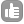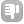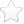0I am relatively new to VNMRJ, and am trying to write a macro that executes an array in experiment 1, then, once finished, executes the array in experiment 2, etc. for any pre-determined number of experiments. My first thought was the following: 1) Start experiment n. 2) Use a "repeat" loop querying the status of the current experiment using acqstatus. 3) When acqstatus indicates that experiment n is complete, exit the "repeat" loop and start the next experiment. The problem that I found is that it was getting stuck in the "repeat" loop, even after the experiment had completed, despite the "until" condition indicating that the loop should be exited once the experiment is complete. I tried a number of different ways of trying to get this to work, but to no avail. My second thought was to try and use the system time to indicate when to start and stop each experiment, however, I cannot find a command/parameter for system time. Is there a command for this? Would I find the macro getting stuck in a loop as with my first approach? Would the pad parameter help me here, where I assign a long enough pre-acquisition delay in the first spectrum of each array from experiment n+1 and onward? (If this is the only way, then so be it, but it would be helpful if the two initial ideas could be done, as I have some applications that would require that they do in the not-so-distant future.). Any help that I can get would be fantastic and very much appreciated. asked Mar 10 '14 at 18:070Hi, This should be straight forward. You can set up the arrays in each experiment and then type "go" in the order that you want to execute them, as VnmrJ queues it up automatically in the order you type "go". For e.g., exp1 to exp10 are set up already with an array, then you can just "jexp1 go", "jexp2 go"... and so on. You can check the number and the order of the queued experiments from the queue in /vnmr/acqqueue/ directory. Or if you are looking for a more sophisticated way of setting them up, then: The one idea that comes to my mind, is to write a macro for the array you want to set up. If all experiments require the same array then one macro should be good (lets call it macro_array, and remember to place it in ~/vnmrsys/maclib). If each experiment requires different arrays, then multiple macros may be required (let the macro names be macro_array_N, N = 1,2,...). Then you can write another macro, where you can create/join experiments and call the macro_array (or macro_array_N), start acquisition, join the next experiment, call macro_array, start acquisition and so on. This will ensure that all parameters that you are trying to keep constant/vary between various experiments are set right. For e.g. (this assumes the experiment numbers already exist, if not you have to create them using cexp(N)). jexp1 macro_array_1 go shell('sleep 10') jexp2 macro_array_2 go shell('sleep 10') .... so on The shell('sleep 10') command will let the VnmrJ terminal sleep for 10s. This might be helpful in certain cases (e.g. multidimensional NMR) where the "go" command might take a few seconds before it has actually queued up a given experiment. I am sure now you would notice that you could also use the sleep command to wait until the previous experiment gets over (provided you can exactly calculate the total measurement time for each experiment). Hope this helps! ~Waj answered Mar 14 '14 at 07:43Bharathwaj 112

 toggle preview

Tags:

×3
×1
×1
×1
×1

Asked: Mar 10 '14 at 18:07

Seen: 2,924 times

Last updated: Mar 14 '14 at 07:43